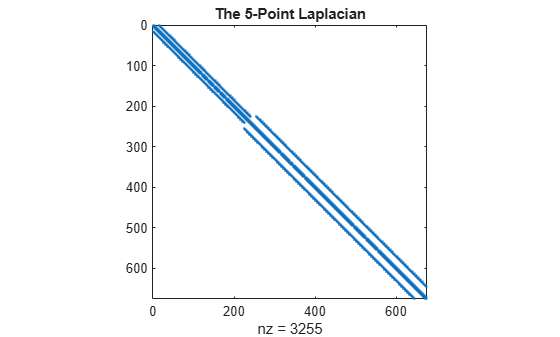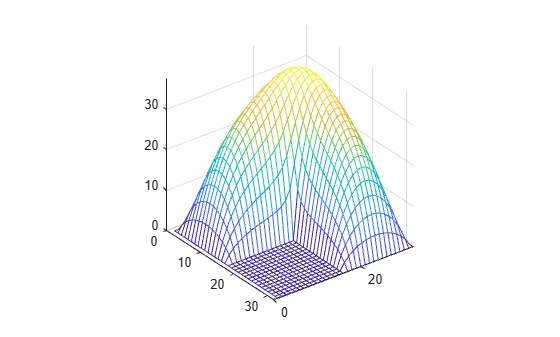# Finite Difference Laplacian

This example shows how to compute and represent the finite difference Laplacian on an L-shaped domain.

### Domain

The `numgrid` function numbers points within an L-shaped domain. The `spy` function is a useful tool for visualizing the pattern of nonzero elements in a matrix. Use these two functions to generate and display an L-shaped domain.

```n = 32; R = 'L'; G = numgrid(R,n); spy(G) title('A Finite Difference Grid')```Show a smaller version of the matrix as a sample.

`g = numgrid(R,10)`
```g = 10×10 0 0 0 0 0 0 0 0 0 0 0 1 5 9 13 17 25 33 41 0 0 2 6 10 14 18 26 34 42 0 0 3 7 11 15 19 27 35 43 0 0 4 8 12 16 20 28 36 44 0 0 0 0 0 0 21 29 37 45 0 0 0 0 0 0 22 30 38 46 0 0 0 0 0 0 23 31 39 47 0 0 0 0 0 0 24 32 40 48 0 0 0 0 0 0 0 0 0 0 0 ```

### Discrete Laplacian

Use `delsq` to generate the discrete Laplacian. Use the `spy` function again to get a graphical feel of the matrix elements.

```D = delsq(G); spy(D) title('The 5-Point Laplacian')```Determine the number of interior points.

`N = sum(G(:)>0)`
```N = 675 ```

### Dirichlet Boundary Value Problem

Solve the Dirichlet boundary value problem for the sparse linear system. The problem setup is:

` delsq(u) = 1` in the interior, `u = 0` on the boundary.

```rhs = ones(N,1); if (R == 'N') % For nested dissection, turn off minimum degree ordering. spparms('autommd',0) u = D\rhs; spparms('autommd',1) else u = D\rhs; % This is used for R=='L' as in this example end```

Map the solution onto the L-shaped grid and plot it as a contour map.

```U = G; U(G>0) = full(u(G(G>0))); clabel(contour(U)); prism axis square ij```Now show the solution as a mesh plot.

```mesh(U) axis([0 n 0 n 0 max(max(U))]) axis square ij```